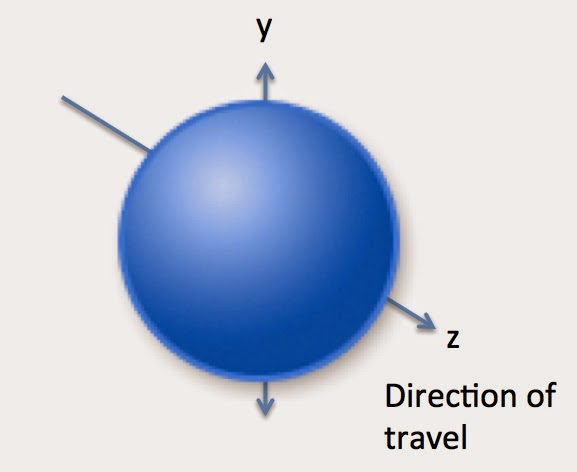### The Higgs field has its own (non-zero) force. It acquires this by a mechanism known as"symmetry breaking" (see previous page).

There are different explanations of how particles might acquire mass (by the Higgs mechanism):

Popular explanation

The simplest explanation, sometimes delivered by physicists pressurised in radio interviews to provide a 30-second explanation, is that the particle (e.g. gauge vector boson) is slowed down by a "treacle-like" or "molasses-like" field around it. This is said to occur in a way perhaps analogous to that in which a politician or pop-star might be slowed down when trying to cross a room while being continually delayed by fans wanting to meet such a famous person. In contrast, another non-famous person might cross more quickly - perhaps analogous to the behaviour of a photon, which does not aquire mass by the Higgs mechanism. Basically, the particles that acquire most mass, are regarded as those that interact most with the Higgs field.

Gaining a "third degree of freedom"

A more detailed explanation is given by Dr Jim Baggott in his book entitled "Higgs" (which contains a foreword by the physics Nobel laureate, Steven Weinberg). In that book, Baggott explains the acquisition of mass as follows: a boson (before interacting with the Higgs field) moves at the speed of light and while travelling (eg in the z direction), can oscillate in the two axes that are perpendicular to that direction (i.e. in the x and y directions i.e. left/right and up/down). It is therefore said to have 2 degrees of freedom. When the boson interacts with the field, it does so by "absorbing" a massless Nambu-Goldstone boson that (as proven by Weinberg, Goldstone & Salam) is created upon the breaking of symmetry [see the previous web-page for symmetry breaking]. The boson that is to gain mass does so by now gaining the ability to oscillate backwards and forwards in the same direction as its direction of travel.

This gain of a "3rd degree of freedom" of movement in the particle's direction of travel (see below) is what causes the particle to slow down and gain energy [and thus gain mass]. It is as if the field is dragging like molasses or treacle on the particle. The degree to which this happens to the particle corresponds to the degree to which it is able to interact with the Higgs field. The mass that it gains is actually inertial mass. Because of its extra oscillations, the particle now has a resistance to acceleration. This is thus equivalent to gaining (inertial) mass. [We know from standard mechanics that the more mass a body has, the more resistance it has to being accelerated (ie it requires more force to achieve the same acceleration - from F=ma].

Baggott himself writes (in chapter 4, page 88): "...Breaking the symmetry creates a massless Nambu-Goldstone boson. This may now be "absorbed" by the massless spin 1 boson to create a third degree of freedom (forward/back). The wave amplitude of the field particle can now oscillate in all three dimensions, including the direction in which it is travelling. The particle acquires "depth" [see the fig below]... In the Higgs mechanism the act of gaining three-dimensionality is like applying a brake. The particle slows down to an extent which depends on the strength of its interaction with the Higgs field.Two degrees of freedom of movement or oscillation of the particle, prior to being given massParticle now has 3rd degree of freedom of movement ie in the same direction as the particle's direction of travel

Above right is an illustration (redrawn from Jim Baggott's) of the gaining of a 3rd "degree of freedom" (i.e. oscillation backwards/forwards in the same direction as the particle is travelling) which is what gives the particle mass. The image on the right is meant to look more ball-like in appearance, to show that the particle now has "depth".

The gaining of this new oscillation (and thus mass) is, at least in some sources, said to be the result of the (initially massless) particle bouncing off Higgs bosons (e.g. see Bojan Golli's online PDF and Minute Physics in Further Reading), with the conversion of some of the particle's initial kinetic energy into "rest energy" mass.

It should be mentioned that although the Higgs field is important for conferring mass to most of the fundamental particles, about 99% of the mass of protons and neutrons (and thus of most of the matter that we know of) is the result of the huge kinetic energy of the gluons and fast-moving quarks, inside the protons and neutrons [and Einstein's energy-mass equivalence].

This kind of movement, eg of the quarks travelling around quickly (and resulting in the mass of protons and neutrons) is different from the smaller scale oscillations (in the direction of travel) that are responsible (according to Jim Baggott (and others: e.g. see the Minute Physics website mentioned in Further Reading) for the (inertial) mass of the quarks themselves.

The maths...

Nicholas Mee's explanation, in the Appendix of his book (see Further Reading), includes something like this:

In the interaction between a force-mediating (or force-carrying) "A" particle (which behaves like a photon but is not a photon itself) and the Higgs field, the total energy of the interaction (between the Higgs field and A) is equal to

A squared x  e squared  x  phi squared

where A represents the force-mediating particle, e is the electric charge of the field particle and
phi is the (non-zero) Higgs field strength (or order parameter). The strength of the interaction is given by e squared.

The amount of mass that is gained by this force-mediating (or force-carrying) "A" particle  upon interacting with the Higgs field is related to the expression:

e squared  x  phi squared

Prof Tom Kibble's explanation:

A far more mathematical explanation is provided by Prof Kibble himself, on Scholarpedia, at:
http://www.scholarpedia.org/article/Englert-Brout-Higgs-Guralnik-Hagen-Kibble_mechanism